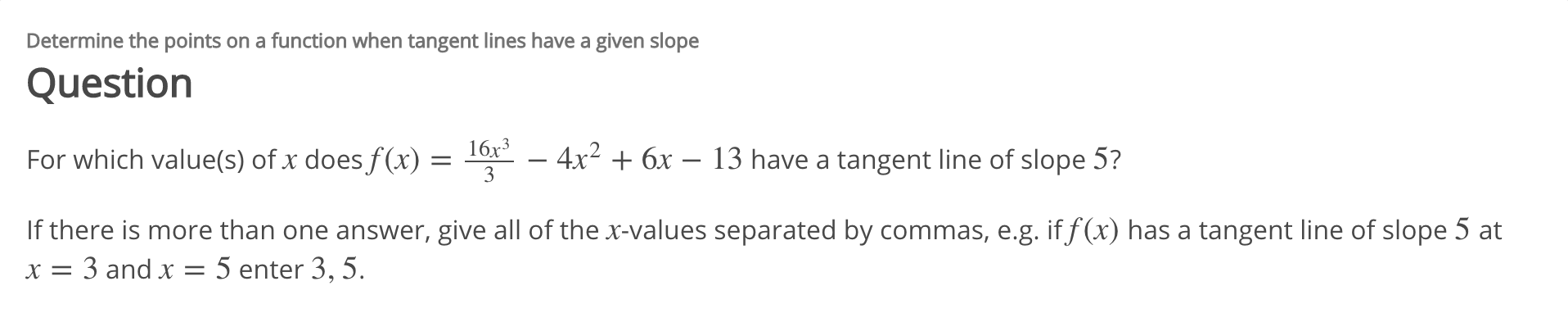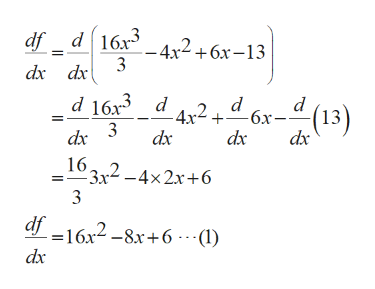# Determine the points on a function when tangent lines have a given slopeQuestion16x3- 4x2 6x - 13 have a tangent line of slope 5?For which value(s) of x does f(x) =3If there is more than one answer, give all of the x-values separated by commas, e.g. if f (x) has a tangent line of slope 5 atx = 3 and x = 5 enter 3, 5.

Question
8 viewshelp_outlineImage TranscriptioncloseDetermine the points on a function when tangent lines have a given slope Question 16x3 - 4x2 6x - 13 have a tangent line of slope 5? For which value(s) of x does f(x) = 3 If there is more than one answer, give all of the x-values separated by commas, e.g. if f (x) has a tangent line of slope 5 at x = 3 and x = 5 enter 3, 5. fullscreen
check_circle

star
star
star
star
star
1 Rating
Step 1

Find value of x for the given function.

Step 2

Differentiate f w.r.t x,help_outlineImage Transcriptionclosedf d16x3 4x2+6x-13 dx dx d d d 163 d -4x2+"6x-"(13) dx 3 dx dx 16 2-4x2x+6 3x2-4x2x+6 3 df -16x2-8x+6---I) dx fullscreen
Step 3

Given: Slope...

### Want to see the full answer?

See Solution

#### Want to see this answer and more?

Solutions are written by subject experts who are available 24/7. Questions are typically answered within 1 hour.*

See Solution
*Response times may vary by subject and question.
Tagged in

### Derivative